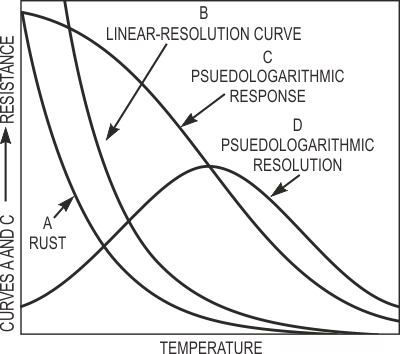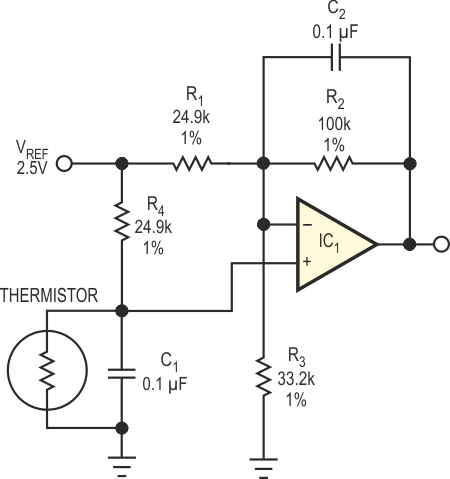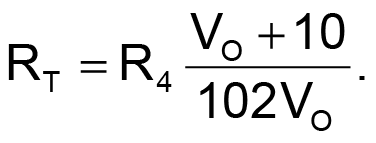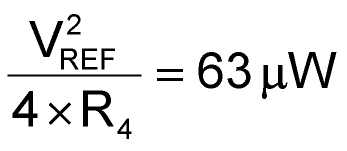# Pseudologarithmic thermistor signal conditioning spans wide temperature range

Stephen Woodward

EDN

Given its low cost, small size, robust construction, accuracy, versatility and sensitivity, it's no wonder that the thermistor rates as one of the most popular temperature sensors available. However, in some applications, a thermistor can exhibit too much sensitivity for wide-range temperature measurements. For example, when you combine a thermistor's radically nonlinear exponential resistance-versus-temperature-response curve with a linear signal conditioner (Reference 1), the resultant graph resembles a difficult-to-characterize response, as Trace A shows (Figure 1).Figure 1. These resistance-versus-temperature curves highlight the improved resolution available in a pseudologarithmic circuit.

Note that most of the thermistor's resistance range crowds into a small span of temperatures at the lower limit of the range. As Curve B in Figure 1 shows, the change of resistance per degree of temperature change looks exaggerated at low temperatures. As temperature increases, the resolution diminishes and may become inadequate at the upper end of the temperature scale.Figure 2. This pseudologarithmic thermistor signal conditioner uses a single operational amplifier and a few passive components.

In contrast, the signal-conditioning circuit in Figure 2 mitigates the thermistor's inherent nonlinearity by generating a compensating, pseudologarithmic response function that's Curve C in Figure 1 represents. The following equation relates the circuit's ±10 V output span for an ADC ±10 V input span to thermistor resistance RT:Curve D in Figure 1 shows the resultant resolution curve. Maximum resolution occurs at a temperature that corresponds to a thermistor resistance equal to R4 and an output voltage of 0 V. Although selection of this value optimizes resolution in the middle of the measurement range, the thermistor's nominal resistance is relatively noncritical. You can also select a different value of reference voltage, VREF, to shift the ±10 V output span to meet other requirements. For best performance, you can share the same reference source for VREF and for the measurement system's ADC, which makes measurements ratiometric and thus insensitive to reference-voltage drift.

In addition to resolution, span, and reference-drift considerations, thermistors – and, for that matter, all temperature sensors – experience self-heating effects. The excitation current produces ohmic power that causes these effects. The limited thermal mass of miniature thermistors provides limited heat dissipation – often only a few microwatts per degree – and that dissipation can produce especially severe temperature shifts.

In the circuit in Figure 2, the power dissipated due to thermistor's self-heating reaches a maximum at midspan – that is, when output voltage is 0 V and equals:for the component values illustrated. For all but the smallest thermistors, this level of self-heating produces acceptably low fractional-degree errors.

EDN